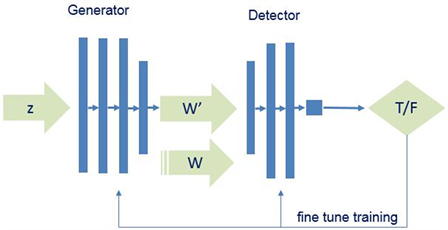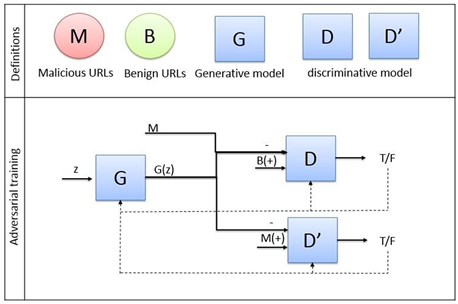﻿ 基于生成式对抗网络的恶意URL数据生成与检测

# 基于生成式对抗网络的恶意URL数据生成与检测Generating and Detection Malicious URL Based on Generative Adversarial Networks

Abstract: Malicious web page recognition based on machine learning is sensitive to data collection and annotation. This paper proposes a method of generating and detecting malicious web pages based on Generative Adversarial Networks (GAN). Design an encoder in order to encode malicious URL at character level. A small number of samples were used to train the model, and the ability of GAN to fit real samples was used to generate malicious web page samples. On the basis of traditional GAN, this paper adds a discriminator to discriminate benign and malignant web pages, and achieves the function of discriminating malicious web pages. Finally, the feasibility of the generated data and the effectiveness of the discriminant model with the currently supervised classifier are verified by vertical and horizontal comparison experiments.

1. 引言

2. 相关工作

2.1. 恶意网页检测

2.2. 生成式对抗网络

GAN的思想来源于博弈论中的纳什均衡 ，也即零和博弈。其模型包含两个部分，分别是生成器(Generator, G)和判别器(Detector, D)。以生成图片为例，G是一个生成网络，它的输入是一个随机噪声z，通过这个噪声生成一个图像W’；而D是一个判别网络，它的任务是判别一张图片是真实的图片还是G生成的图片。D的输入是真实图片W和G生成的图片W’，D通过输出一个概率来判定图片的真实性，如D输出结果是1，那图片则来自真实图片，如果输出结果为0，则图片来自于G成生的图片。在训练过程中，G的任务是尽可能的学习图片的特征骗过D，使D对W’的判定值越高越好。而D则尽可能的提高自己的判别能力，以免被G欺骗。G和D构成了一个动态的“博弈过程”，最终的平衡点即纳什均衡点。对应的GAN生成网络模型如图1所示。Figure 1. GAN model schematic diagram

3. 恶意URL字符生成判别模型

3.1. 域名字符分析

URL (Uniform Resource Locator)统一资源定位符，它表示某一网络资源存在于所在计算机网络上的位置，同时也是浏览器用于检索web上公布的任何资源的机制。我们对URL编码可以采取One-Hot编码方式，这是一种基础的一位有效位编码方式。类似一种将词库的所有词和每种状态对应的编码，是一种全映射的关系。但是这种方法存在明显的缺点，其一，该表示方法看不出词和词直接的关联，是一种任意表示，其二，如果词汇表的数量过于庞大时，那么这种表示方法势必会造成维度灾难。

3.2. 编码器

${A}^{\prime }\left({u}_{i}\right)=\frac{A\left({u}_{i}\right)-MINA}{MAXA-MINA}$ (1)

$\text{A}\left(\stackrel{\to }{u}\right)=\left[119,119,119,46,98,97,105,100,117,46,99,111,109\right]$ ;

${\stackrel{\to }{u}}^{\prime }=\left[0.914,0.9140.914,0.138,0.691,0.680,0.765,0.712,0.893,0.138,0.702,0.829,0.808\right]$

URL的长度都是长短不一的，但大部分URL的长度都不超过200个字符，故在此设计的URL向量的维度为200，对于一些维度不足的URL，在向量后采取补0操作。在所有URL映射为200维的向量之后，就可以将其作为生成式对抗网络的训练数据了。

3.3. 模型设计Figure 2. Framework of MU-GAN

3.4. 损失函数

$\begin{array}{c}\underset{D}{\mathrm{max}}V\left(D,{D}^{\prime },G\right)={\text{Ε}}_{b~{P}_{data}\left(b\right)}\left[\mathrm{log}\left(D\left(b\right)\right)\right]+{\text{Ε}}_{z~{P}_{z}\left(z\right)}\left[\mathrm{log}\left(1-D\left(G\left(z\right)\right)\right)\right]\\ +{\text{Ε}}_{m~{P}_{data}\left(m\right)}\left[\mathrm{log}\left(1-D\left(G\left(z\right)\right)\right)\right]\end{array}$ (2)

$\underset{{D}^{\prime }}{\mathrm{max}}V\left(D,{D}^{\prime },G\right)={\text{Ε}}_{m~{P}_{data}\left(m\right)}\left[\mathrm{log}\left({D}^{\prime }\left(m\right)\right)\right]+{\text{Ε}}_{z~{P}_{z}\left(z\right)}\left[\mathrm{log}\left(1-{D}^{\prime }\left(G\left(z\right)\right)\right)\right]$ (3)

$\underset{G}{\mathrm{min}}V\left(D,{D}^{\prime },G\right)={\text{Ε}}_{z~{P}_{z}\left(z\right)}\left[\mathrm{log}\left(1-{D}^{\prime }\left(G\left(z\right)\right)\right)\right]+{\text{Ε}}_{z~{P}_{z}\left(z\right)}\left[\mathrm{log}\left(1-D\left(G\left(z\right)\right)\right)\right]$ (4)

4. 实验分析

4.1. 实验环境与数据集Table 1. Experimental environment configuration

4.2. 实验设计Table 2. Generative network’s parameters configurationTable 3. Discriminator network D parameters configurationTable 4. Discriminator network D’ parameters configuration

4.3. 实验结果分析Table 5. The accuracy of three classifiers in five sets of data (%)Table 6. The comparison of four classifier (%)

5. 结束语

 中国互联网信息中心. 第44次中国互联网发展状况统计报告[EB/OL]. http://www.cac.gov.cn/2019-08/30/c_1124938750.htm, 2019-08-30

 瑞星. 2019年中国网络安全报告[EB/OL]. http://it.rising.com.cn/dongtai/19692.html, 2020-01-15

 赛门铁克. 2018年互联网安全威胁报告[EB/OL].
https://www.symantec.com/security-center/threat-report, 2019-04

 沙泓州, 刘庆云, 柳厅文, 周舟, 郭莉, 方滨兴. 恶意网页识别研究综述[J]. 计算机学报, 2016, 39(3): 529-542.

 Goodfellow, I.J., Bengio, Y. and Cour-ville, A. (2017) Deep Learning. MIT Press, Cambridge, 1-3.

 Goodfellow, I.J., Pouget-Abadie, J., Mirza, M., et al. (2014) Generative Adversarial Nets. Advances in Neural Information Processing Systems 27: Annual Conference on Neural Information Processing Systems, Montreal, 8-13 December 2014, 2672-2680.

 Prakash, P., Kumar, M., Kompella, R.R., et al. (2010) PhishNet: Predictive Blacklisting to Detect Phishing Attacks. Proceedings of the 29th IEEE International Conference on Computer Communications, San Diego, 15-19 March 2010, 1-5.

 Sahoo, D., Liu, C.H. and Hoi, S.C.H. (2017) Malicious URL Detection Using Machine Learning: A Survey.

 Moshchuk, A., Bragin, T., Deville, D., et al. (2007) Execution-Based Detection of Malicious Web Content. Proceedings of 16th USENIX Security Symposium, Boston, 3:1-3:16.

 Rieck, K., Krueger, T. and Dewald, A. (2010) Efficient Detection and Prevention of Drive-by-Download Attacks. Proceedings of the 26th Annual Computer Security Applications Conference, Austin, 6-10 December 2010, 31-39.

 Tobiyama, S., Yamaguchi, Y., Shimada, H., et al. (2016) Malware Detection with Deep Neural Network Using Process Behavior. Proceedings of the 40th Annual Computer Software and Applications Confer-ence, Atlanta, 10-14 June 2016, 577-582.
https://doi.org/10.1109/COMPSAC.2016.151

 张洋, 柳厅文, 沙泓州, 时金桥. 基于多元属性特征的恶意域名检测[J]. 计算机应用, 2016, 36(4): 941-944 + 984.

 Rumelhart, D.E., Hinton, G.E. and Williams, R.J. (1986) Learning Representations by Back-Propagating Errors. Nature, 323, 533-536.
https://doi.org/10.1038/323533a0

 He, D., Chen, W., Wang, L., et al. (2013) A Game-Heoretic Ma-chine Learning Approach for Revenue Maximization in Sponsored Search. In: International Joint Conference on Artifi-cial Intelligence, AAAI Press, Beijing, 206-212.

 王坤峰, 苟超, 段艳杰, 等. 生成式对抗网络GAN的研究进展与展望[J]. 自动化学报, 2017, 43(3): 321-332.

 Srivastava, N., Hinton, G., Krizhevsky, A., et al. (2014) Drop-out: A Simple Way to Prevent Neural Networks from Overfitting. Journal of Machine Learning Research, 15, 1929-1958.

Top